Parenting

# How to Help Students Understand Math Better?

1.5k views

 1 Introduction 2 8 Ways to Help Students Understand Math 3 Conclusion 5 Frequently Asked Questions (FAQs) 6 External References

23 May 2017

## Introduction

Math always seems like a tough ordeal, more for the teachers who teach than for the students who learn the subject. Math is based on logic and rules that you can study and apply – there isn’t any special skill involved.

Working on math every day is the real key to understanding it. The more time you spend around the number and concepts the more you become thorough with the subject in order to understand math.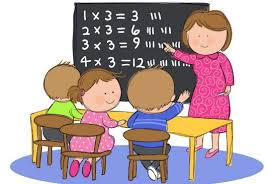Considering Math is a life skill rather than how it’s perceived today, as a school subject, wouldn’t we want students to understand math?

To make kids understand math, effective teaching practice can be learned.

If you ever want to read it again as many times as you want, here is a downloadable PDF to explore more.

## 8 Ways to Help Students Understand Math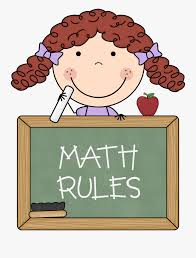### 1. Do you encourage your student's mistakes?

The first and foremost step is to encourage mistakes. This is a sign of active learning. When a student works out a problem and makes mistakes, she is showing far more progress than just listening to a lecture about that very concept.

Ensuring that she understands her mistakes and corrects them on her own is a crucial part of her learning process.Math has always been about practice, never about definitions or formulas and the Cuemath Learning System makes sure of this. Read more about Cuemath Learning Methodology.

### 2. Do you encourage questions from your students?

Children are naturally curious. A question is a sign of curiosity. Set aside some time and encourage students to ask questions. This ensures that their curiosity is ignited at all times.

Focus on showing your students that questions matter by giving encouraging responses.

Moreover, learning the “why” behind the “what” of every concept is crucial to imbibe the concept. Encourage an atmosphere like this. You will find that students themselves begin to answer questions of their peers.### 3. How to understand math by using physical learning aids?

Physical learning aids are considered a blessing for students. Stanford education professor Jo Boaler explains that when children count on their fingers, they are building their mathematical ability.

Similarly, learning aids can help students develop a strong foundation in various concepts. The most common example of this is the abacus counting frame that helps children count.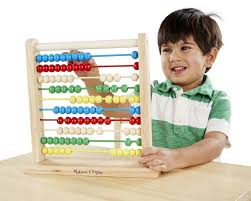Cuemath Teachers are equipped with Cuemath’s Mathbox that consists of a number of learning aids that help students discover math better. Watch the video given below to see how students are benefitted from physical learning aids.

### 4. How to understand math by using visual models?

Once students have learned the basics with physical learning aids, their skills can be scaled using visual models to deepen their understanding.

For example, showing a child one grape next to a bunch of 20 grapes will help them analyze that the number 20 is much bigger than the number 1. Similarly, even concepts of fractions, algebra, geometry, etc. can be made easy using visual methods.

Once a student is able to “see” math, it will be easier to introduce him/her to the more abstract concepts of math. Watch the video given below to see how visual modeling helps students understand math.

### 5. Do you give your student time-based challenges?

Engage students by allowing them to play time-based games online or on math gaming apps. Games like these help children build their aptitude and improve individual skills like agility, pattern recognition, and many more.

Moreover, technology is an important facet in today’s world and it hooks them on for a very long time.

Cuemath Teachers use the Cuestudent App to help students build their aptitude with the vast number of delightful games and exercises on it, specially designed for each grade.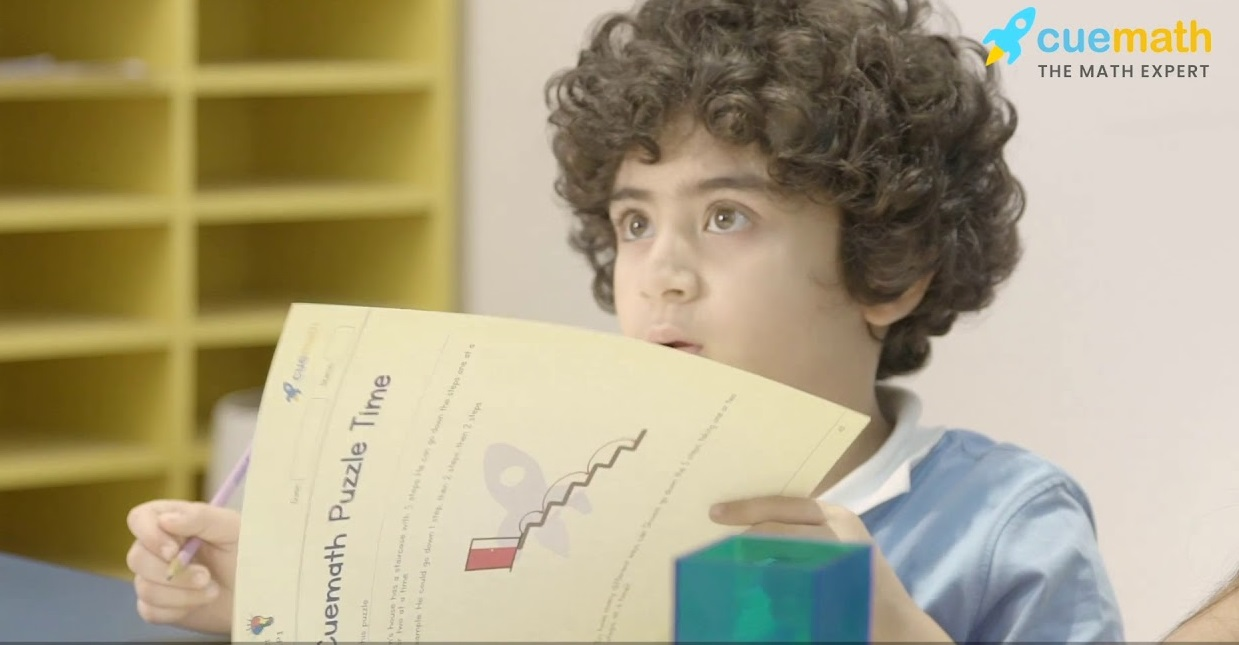### 6. How do puzzles help students understand math?

Introducing puzzles to students as a method of learning helps them devise their own ways around problems and concepts. This improves their reasoning skills to a large extent.

Allow them to work individually or in groups and be open to multiple answers. As far as puzzles go, there may be more than one right answer!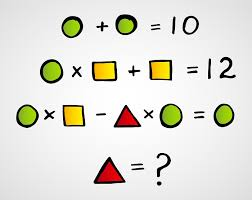The Creative Reasoning Cards by Cuemath are carefully designed so as to widen a student’s understanding of concepts and go above and beyond it. Read Math Puzzles for Class 3

### 7. Put in Some Math Games

Most math classes spell boredom for students and they look for ways to skip it. This doesn’t have to be the case if math games are brought into the picture.

Games like Ken-Ken, Sudoku, and some other popular board games available in the market increase the interest among students.

Cuemath employs many such Math Games to not only increase interest among students but also to impart knowledge.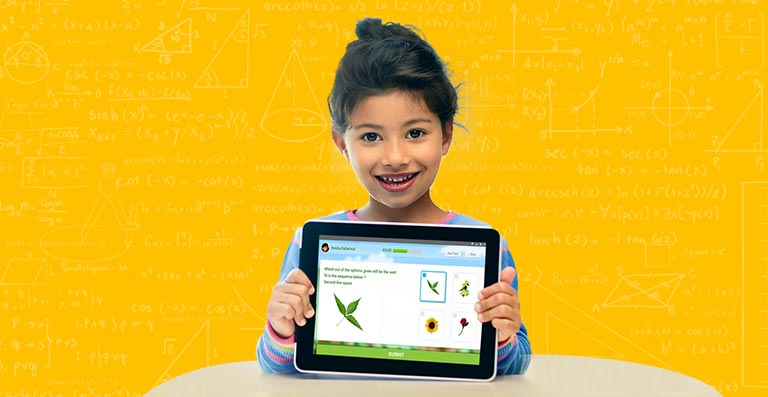### 8. Apply Math to Real-World Problems

Any subject would seem redundant to students if they don’t understand it’s used in everyday life. This is where theory and practical knowledge combine.

When students are able to gauge priorities and employ those decision-making skills, they are practically using math.## Conclusion

The ultimate goal of teachers is to help students understand the subject. To make kids understand math, the effective teaching methods mentioned above have to be adopted.

Connecting with your students and using research-based strategies will help them understand the subject better. Here are a few tips to help students understand the subject better;

• Making kids practice math problems
• Conducting formative assessments
• Connecting math to other learning areas
• Encouraging math games and puzzles

Cuemath’s workbooks tackle problems wherein the word problems given to the students help them grasp the practical usage of a particular concept.

Cuemath, a student-friendly mathematics and coding platform, conducts regular Online Live Classes for academics and skill-development, and their Mental Math App, on both iOS and Android, is a one-stop solution for kids to develop multiple skills. Understand the Cuemath Fee structure and sign up for a free trial.

## 1. How to help students understand math?

Following are the strategies to help students understand math:

• Making kids practice math problems
• Conducting formative assessments
• Connecting math to other learning areas
• Encouraging math games and puzzles

## External References

Related Articles
Award-winning math curriculum, FREE for a year